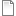# Figure 5.15Features of a two-state kinetic scheme

Features of a two-state kinetic scheme. (a) Simulated sample of the time course of channel conductance of the kinetic scheme described in Scheme (5.13). The parameters are α = 1 ms-1 and β = 0.5 ms-1. (b) Histogram of the open times in a simulation and the theoretical prediction of 0.5e-0.5t from Equation 5.14. (c) Histogram of the simulated closed times and the theoretical prediction e-t.

Simulation environment:
Notes

To run the simulation:

1. In the Run Control window click on Init & Run
2. In the top graph a trace of the conductance of the channel should appear. In the middle and lower graphs  histogram of the open and closed time distributions with theoretical predictions should appear.

To change the rate of the opening and closing:

1. In the ChannelBuild window click on O: 2 state, 1 transitions
2. In the ChannelBuildGateGUI window that opens, change the values in the boxes at the lower right. The forward rate (α in the figure) is aCO and the backard rate β is aCO.fig-5-15.nrnzip